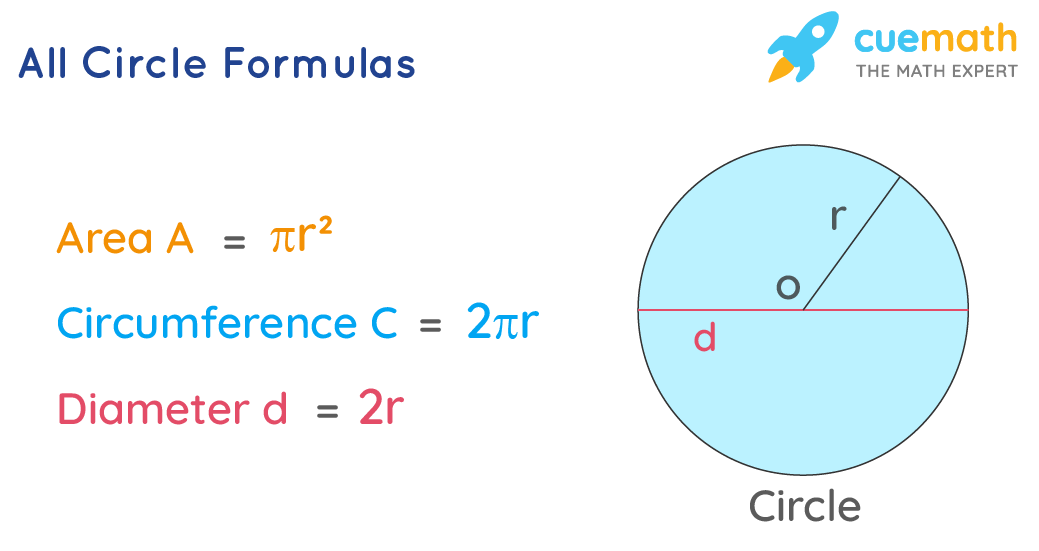# All Circle Formulas

Go back to  'Math Formulas'

The circle is defined as the set of points placed at equal distance from a single point in a plane. The midpoint of the circle is called the center of the circle. Parameters like area, circumference, the radius of a circle can be calculated using all circle formulas.

Let us understand all circle formulas using solved examples.

## What Are All Circle Formulas?

Different Circle Formulas to calculate different parameters of a given circle can be expressed as,The diameter of a Circle D = 2 × r

Circumference of a Circle C = 2 × π × r

Area of a Circle    A = π × r2

Where,

r = radius of the circle

d = diameter of the circle

c = circumference of the circle

## Solved Examples Using All Circle Formulas

### Find the area of the circular park whose radius is equal to 200m.

Solution:

To find: Area of a park.

Given:

The radius of the park = 200 m

Using Area of a Circle Formula,

Area of a Circle = π × r2

= π × 2002

= π × 40000

Answer:  The area of the circular park is 40000π m2.

### If the radius of a circle is 7 units. then find the circumference of a circle.

Solution:

To find: circumference of a circle

Given:

The radius of circle = 7 units

Using the circumference formula,

Circumference of a Circle, C = 2 ×  π ×  r

= (2 × π  × 7) units

C = 14π

Answer: The circumference of the circle is 14π units.